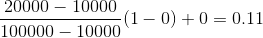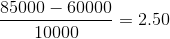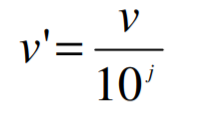Data Transformation in Data Mining

• Last Updated : 03 Feb, 2020

The data are transformed in ways that are ideal for mining the data. The data transformation involves steps that are:

1. Smoothing:
It is a process that is used to remove noise from the dataset using some algorithms It allows for highlighting important features present in the dataset. It helps in predicting the patterns. When collecting data, it can be manipulated to eliminate or reduce any variance or any other noise form.

The concept behind data smoothing is that it will be able to identify simple changes to help predict different trends and patterns. This serves as a help to analysts or traders who need to look at a lot of data which can often be difficult to digest for finding patterns that they wouldn’t see otherwise.

2. Aggregation:
Data collection or aggregation is the method of storing and presenting data in a summary format. The data may be obtained from multiple data sources to integrate these data sources into a data analysis description. This is a crucial step since the accuracy of data analysis insights is highly dependent on the quantity and quality of the data used. Gathering accurate data of high quality and a large enough quantity is necessary to produce relevant results.

The collection of data is useful for everything from decisions concerning financing or business strategy of the product, pricing, operations, and marketing strategies.

For example, Sales, data may be aggregated to compute monthly& annual total amounts.

3. Discretization:
It is a process of transforming continuous data into set of small intervals. Most Data Mining activities in the real world require continuous attributes. Yet many of the existing data mining frameworks are unable to handle these attributes.

Also, even if a data mining task can manage a continuous attribute, it can significantly improve its efficiency by replacing a constant quality attribute with its discrete values.

For example, (1-10, 11-20) (age:- young, middle age, senior).

4. Attribute Construction:
Where new attributes are created & applied to assist the mining process from the given set of attributes. This simplifies the original data & makes the mining more efficient.

5. Generalization:
It converts low-level data attributes to high-level data attributes using concept hierarchy. For Example Age initially in Numerical form (22, 25) is converted into categorical value (young, old).

For example, Categorical attributes, such as house addresses, may be generalized to higher-level definitions, such as town or country.

6. Normalization: Data normalization involves converting all data variable into a given range.
Techniques that are used for normalization are:

• Min-Max Normalization:
• This transforms the original data linearly.
• Suppose that: min_A is the minima and max_A is the maxima of an attribute, P

We Have the Formula:• Where v is the value you want to plot in the new range.
• v’ is the new value you get after normalizing the old value.

Solved example:
Suppose the minimum and maximum value for an attribute profit(P) are Rs. 10, 000 and Rs. 100, 000. We want to plot the profit in the range [0, 1]. Using min-max normalization the value of Rs. 20, 000 for attribute profit can be plotted to:And hence, we get the value of v’ as 0.11

• Z-Score Normalization:
• In z-score normalization (or zero-mean normalization) the values of an attribute (A), are normalized based on the mean of A and its standard deviation
• A value, v, of attribute A is normalized to v’ by computingFor example:
Let mean of an attribute P = 60, 000, Standard Deviation = 10, 000, for the attribute P. Using z-score normalization, a value of 85000 for P can be transformed to:And hence we get the value of v’ to be 2.5

• Decimal Scaling:

• It normalizes the values of an attribute by changing the position of their decimal points
• The number of points by which the decimal point is moved can be determined by the absolute maximum value of attribute A.
• A value, v, of attribute A is normalized to v’ by computing• where j is the smallest integer such that Max(|v’|) < 1.

For example:

• Suppose: Values of an attribute P varies from -99 to 99.
• The maximum absolute value of P is 99.
• For normalizing the values we divide the numbers by 100 (i.e., j = 2) or (number of integers in the largest number) so that values come out to be as 0.98, 0.97 and so on.
My Personal Notes arrow_drop_up